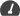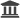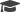## About the Course

Syllabus Unit I: Role of Statistics in Engineering. Over view of Engineering Data Collection, Random experiments, sample spaces, events, probability measure on events definition, properties, examples. Conditional probability definition, properties, examples, Bayes theorem, independent events. Unit II: Definition of random variables, standard discrete and continuous random variables -viz. Bernoulli, Binomial, Geometric, Poisson, Exponential, Gamma, Normal. Expectation, variance, other properties. Unit III: Definition of bivariate random variables, joint distributions, covariance and correlation between two random variables, independence, distributions of sums. Unit IV: Data collection methods, types of data, graphical summaries of data, numerical summaries of univariate data, bivariate summaries,measures of association. Unit V: Introduction to statistical inference, population parameters, variable(s) of interest, statistic, estimators as random variables. Douglas C. Montgomery George C. Runger Applied Statistics and Probability for Engineers Wiley ISV Paperback – 1 January 201603:00UoHMathematicsENNot StartedFrom: 19/08/2020 To: 30/12/2020

## Course Instructors